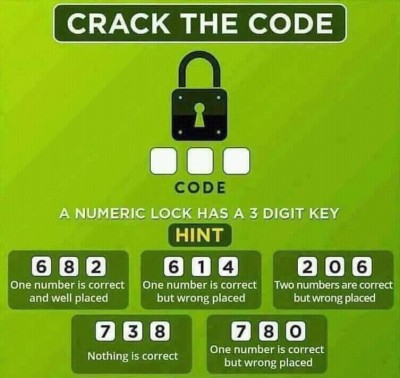### Crack The Code

Follow the hint strickly and see, if you can crack this code.#### Related Posts

###### Nigeria Does not Determine WAEC Timetable - PTF Cordinator

026
Follow
It's 062. I dont think it could be 026 because the three correct numbers are 0 2 6 in numerical order as the 2nd row 1st column box says 7, 3 and 8 are all incorrect but if you look at the first row 2nd column box it says one is correct but wrongly placed so 6 isnt the first digit and then look at the first row first column box it says one is correct and well placed. We know it's not talking about 6 because the first row 2nd column box already contradicts that so it is talking about 2. Then to get the full code you look at the first row third column box its says two numbers are correct but wrongly placed. Place the 2 gotten from the first row first column box u have - - 2 then from then from the information in the first row 2nd column box u know that 6 cannot be the first digit of the code , therefore the code is 062.
Follow
• ibrahimmuhd: 6 will never be the code digit because from the first row containing 682 the hint say one number is correct and well placed not two numbers which u got it as I do thats no 2. If that is the case then there is mo way 6 or 8 could be among the code digits.
2 years ago
Follow
056 is the code
Follow
Hurray!
042 is correct!
Follow
062 is the code
Follow
862
Follow
062
Follow
780 is the correct code
Follow
It's 102
Follow
@myschool should stop wasting our time and tell us the answers to these questions. i still dont know the correct answer to the last riddle
Follow

#### Quick Questions

• 2 Answers   ·   1 hour ago
• 3 Answers   ·   2 hours ago
• 2 Answers   ·   10 hours ago

#### Post Comment

Please don't post or ask to join a "Group" or "Whatsapp Group" as a comment. It will be deleted. To join or start a group, please click here

It's 062. I dont think it could be 026 because the three correct numbers are 0 2 6 in numerical order as the 2nd row 1st column box says 7, 3 and 8 are all incorrect but if you look at the first row 2nd column box it says one is correct but wrongly placed so 6 isnt the first digit and then look at the first row first column box it says one is correct and well placed. We know it's not talking about 6 because the first row 2nd column box already contradicts that so it is talking about 2. Then to get the full code you look at the first row third column box its says two numbers are correct but wrongly placed. Place the 2 gotten from the first row first column box u have - - 2 then from then from the information in the first row 2nd column box u know that 6 cannot be the first digit of the code , therefore the code is 062.
Abdulwarrior

2 Members
7 Members
• 21st
Jul 2020
##### Marketplace
• Here are the things students around you have to sell;
• ###### Samsung galaxy Grand prime+
₦36,000   Used
• ###### Digital SLR Camera Sony HX 200V
₦70,000   OFFAPOLY   Used